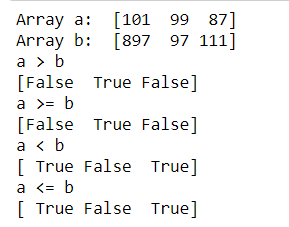Related Articles
How to compare two NumPy arrays?
• Last Updated : 01 Oct, 2020

This article focuses on the comparison done using NumPy on arrays. Comparing two NumPy arrays determines whether they are equivalent by checking if every element at each corresponding index are the same.

Method 1:

We generally use the `==` operator to compare two NumPy arrays to generate a new array object. Call ndarray.all() with the new array object as ndarray to return True if the two NumPy arrays are equivalent.

 `import` `numpy as np`` ` `an_array ``=` `np.array([[``1``, ``2``], [``3``, ``4``]])``another_array ``=` `np.array([[``1``, ``2``], [``3``, ``4``]])`` ` `comparison ``=` `an_array ``=``=` `another_array``equal_arrays ``=` `comparison.``all``()`` ` `print``(equal_arrays)`

Output:

`True`

Method 2:

We can also use greater than, less than and equal to operators to compare. To understand, have a look at the code below.

```Syntax : numpy.greater(x1, x2[, out])
Syntax : numpy.greater_equal(x1, x2[, out])
Syntax : numpy.less(x1, x2[, out])
Syntax : numpy.less_equal(x1, x2[, out])```
 `import` `numpy as np`` ` ` ` `a ``=` `np.array([``101``, ``99``, ``87``])``b ``=` `np.array([``897``, ``97``, ``111``])`` ` `print``(``"Array a: "``, a)``print``(``"Array b: "``, b)`` ` `print``(``"a > b"``)``print``(np.greater(a, b))`` ` `print``(``"a >= b"``)``print``(np.greater_equal(a, b))`` ` `print``(``"a < b"``)``print``(np.less(a, b))`` ` `print``(``"a <= b"``)``print``(np.less_equal(a, b))`

Output:Attention geek! Strengthen your foundations with the Python Programming Foundation Course and learn the basics.

To begin with, your interview preparations Enhance your Data Structures concepts with the Python DS Course.

My Personal Notes arrow_drop_up